# Printable Math Games## Upper Elementary & Middle School Math Games

### Math Teachers, Look No Further! 42 printable math games for upper elementary and middles school students, easy-to-setup for any math class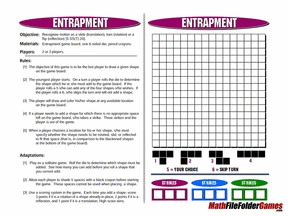#### Get 3 Free Samples (CLICK ON THE “PREIEW” BUTTON):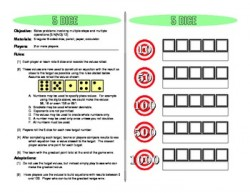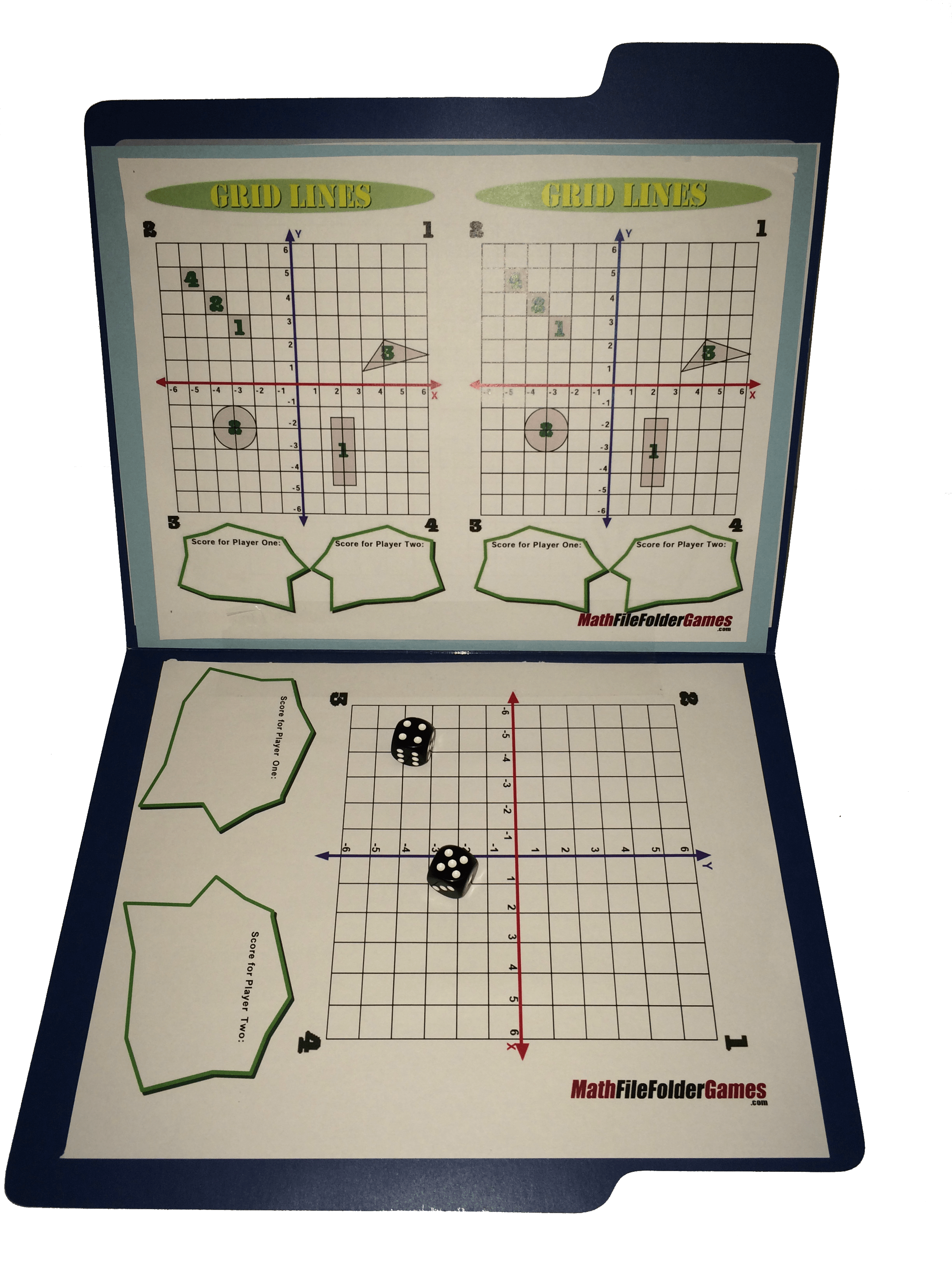What is a Math File Folder Game?
A Math File Folder Game fits in a folder making it easy to use and ready at a moment’s notice. Very few other items are required. You’ll need to construct or reproduce the included game boards and have playing cards and dice handy. A few games use other types of geometric manipulatives that are standard items in most elementary and middle school classrooms.

#### Here’s what some teachers have said so far:

I feel like I just hit the jack pot! Thank you for this great resource! – Hodges Herald

I’m soo glad to find folder games for middle school! – Hannah Wyss

Contains some great resources for intermediate math centers! – Isabel Loveluck

Wow, what a great deal! The games are fantastic and simple to learn! – Teaching the Gifted

I have only used three of the games from this pack and my kids ask for more. So much fun and learning wrapped into one product! Well done and thank you! – Teachercynzg

I am so glad to see these for older students! Thank you so much for sharing. – Amy Allen

This is an awesome resource! – menordman

The games have been a great hit with the class. Many thanks! – amberlo0

Thank you so much for creating such a wonderful resource! Great way for the kids to review concepts. – mrspjam

Excellent resource and a lot of fun for the students. – ebrance

Thanks for making up these games. It has saved me many hours of making learning fun for the students. – 1234n

I was looking for games for my high kiddos as I have lots of games for my on grade level and below. This fit my needs perfectly! Thank you! – kwong331This value-packed book contains 42 math games that will give your students so much fun they’ll forget they’re actually learning mathematics! But don’t be fooled. Just because games are exciting doesn’t mean that they’re not built upon solid educational benefits.

#### Here are ten benefits that these great Math File Folder Games provide:

1. Children are motivated to play games so they choose to actively participate and enjoy playing. While they’re playing, learning comes easier to them.
2. Games provide engaging ways to apply the new mathematical skills they’re learning.
3. Because these games reduce the fear of failure, they help kids build their math self-confidence and reduce their math anxiety.
4. Games provide opportunities for trying out different types of problem-solving strategies and for increasing number sense.
5. Compared to more formal types of classroom activities, games provide an environment where children can interact with each other and increase their abilities to work and play as a team.
6. Games give students ways to interact at different levels of ability. One child might be just learning a new concept, another may be deepening his or her understanding, a third might be synthesizing previously learned concepts, and a fourth might be reviewing prerequisites for upcoming materials.
7. As a teacher or parent observes students playing and making choices, he or she can detect areas where students are having difficulty with specific concepts.
8. Math games give students the chance to play hands-on as they interact with manipulatives that will help them to understand mathematical principles more readily.
9. By working independently of the teacher, students use the rules of the game and their own motivation to stay focused to achieve their goal–winning the game!
10. The basic architecture of these math games is common to so many cultures that children who are struggling with their English language skills can still participate.

In addition to all these great benefits, kids will learn basic skills, problem solving, and critical thinking across a wide variety of mathematical topics: measurement, arithmetic, algebra, and geometry.

#### Here are some of the specific topics covered:

congruence, similarity, perimeter, area, volume, linear measurement in standard and metric units, generating and analyzing patterns and relationships, practice and strategy with the four basic operations, understanding of fractions, both equivalence and ordering, modeling with mathematics, relationships between 2-D and 3-D objects, probability models, greatest common factor, least common multiple, prime factorization, ratio, proportion, and percent, liquid volumes, classification of angles, graphing on a coordinate plane, complementary and supplementary angles, rules for divisibility, operations with integers and rational numbers, absolute value, integer exponents, radicals, one variable equations, Pythagorean Theorem, statistical variability and measures of central tendency

Math File Folder Games also offer teachers another benefit. They align to the US Common Core Standards and Common Core Mathematical Practices.

How to Make Games Successful in Your Classroom

• Choose games that match your current classroom objectives.
• Don’t just use games to fill time. Integrate them as part of your regular lesson planning.
• Customize the game to fit the level of your students and then revise the rules to challenge them. This book includes adaptation ideas for all 42 games.
• Pick one of the simpler games to send home as part of a regular homework assignment.
• Try different numbers of players–two, four, and team groups.

By using Math File Folder Games at home or in your classroom with your upper elementary, middle school, or high school kids, you can provide them with hours of fun-filled learning time. And the best part is you’ll get into the spirit and enjoy yourself, too!## 42 Middle School Math Games

“How did I every teach without these! Thanks for creating games that make my students smile when they practice their math skills.” – DONDRA P.

“This is a great resource. I was able to find games that supported both my 7th and 8th grade math classes!” – Emily D.

“My kids loved the few games I purchased so much, I decided to just get the lot. I gave out copies to pairs of kids and they rated which ones they wanted to do first. We now have File Folder Friday” – Susan M.

“I love this, I’m always looking for games that reinforce skills for my struggling learners. I use them for stations. Thanks!” – Karen C.

This is a great assortment of games that are fast and two the point and provide conversation between students about the topic. – tejackson

EXCELLENT resource! Each game is quick and easy- can be done as a anticipatory activity and/or closing activity. Thank you! – Karen Vallone

Students really enjoyed using these games. Have only used a few but the overall response was 100% positive. – Brianna B

# 42 Printable Math Games

## Common Core Mathematical Standard

Note: Most of these math games can be modified and used with different grade levels.#### (1) Entrapment

8.G Understand congruence and similarity using physical models, transparencies, or geometry software.
8.G.1
8.G.2
8.G.3

#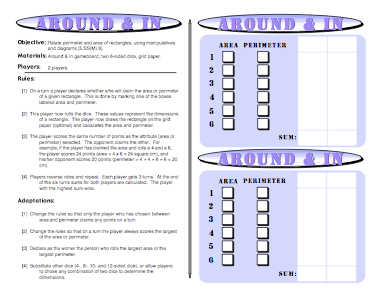#### (2) Around and In

3.MD
Geometric measurement: recognize perimeter as an attribute of plane figures and distinguish between linear and area measures.

8. Solve real world and mathematical problems involving perimeters of polygons, including finding the perimeter given the side lengths, finding an unknown side length, and exhibiting rectangles with the same perimeter and different areas or with the same area and different perimeters.

####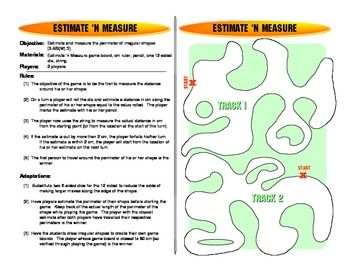(3) Estimate ‘N Measure

1.MD Measure lengths indirectly and by iterating length units.

2.MD Measure and estimate lengths in standard units.

Common Core Standards for Mathematical Practice
5. Use appropriate tools strategically.

####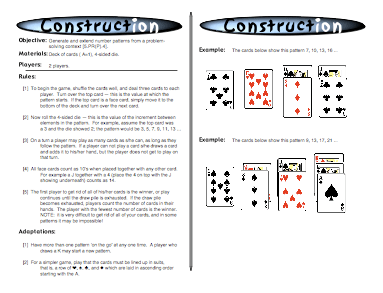(4) Construction

4.OA Generate and analyze patterns.
5. Generate a number or shape pattern that follows a given rule. Identify apparent features of the pattern that were not explicit in the rule itself. For example, given the rule “Add 3” and the starting number 1, generate terms in the resulting sequence and observe that the terms appear to alternate between odd and even numbers. Explain informally why the numbers will continue to alternate in this way.

#### (5) 5 Dice

3.OA Solve problems involving the four operations, and identify and explain patterns in arithmetic.

3.NBT Use place value understanding and properties of operations to perform multi-digit arithmetic.

5.OA Write and interpret numerical expressions.

Common Core Standards for Mathematical Practice.
1. Make sense of problems and persevere in solving them.

####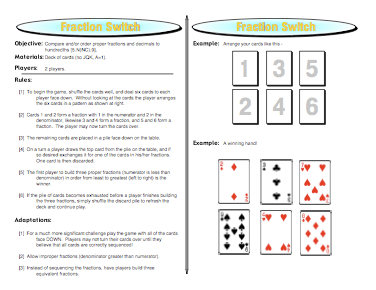(6) Fraction Switch

3.NF Develop understanding of fractions
as numbers.

4.NF Extend understanding of fractional equivalence and ordering.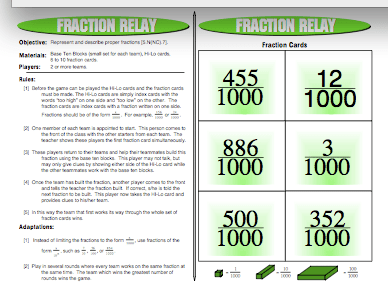#### (7) Fraction Relay

3.NF Develop understanding of fractions
as numbers.

4.NF Extend understanding of fractional
equivalence and ordering.

Common Core Standards for Mathematical Practice
4. Model with Mathematics

####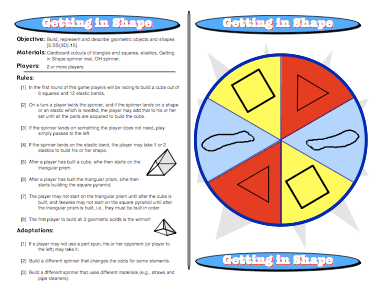(8) Getting in Shape

G-GMD Visualize relationships between two-dimensional and three- dimensional objects.

Common Core Standards for Mathematical Practice
4. Model with Mathematics

####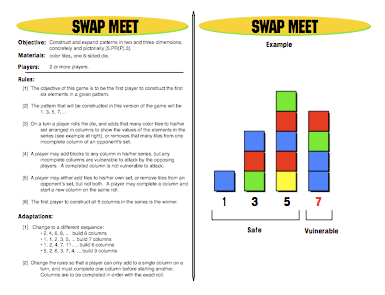(9) Swap Meet

4.OA Generate and analyze patterns.

Common Core Standards for Mathematical Practice
7. Look for and make use of structure.

####(10) Spin to Win

7.SP Investigate chance processes and develop, use, and evaluate probability models.

Common Core Standards for Mathematical Practice
4. Model with Mathematics

####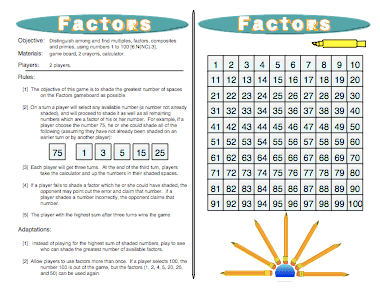(11) Factors

6.NS.4 Compute fluently with multi-digit numbers and find common factors and multiples.

Common Core Standards for Mathematical Practice
1. Make sense of problems and persevere in solving them.
7. Look for and make use of structure.

####(12) Boxes

7.G Solve real-life and mathematical problems involving angle measure, area, surface area, and volume.

Common Core Standards for Mathematical Practice
4. Model with mathematics.

####(13) Half N Half

3.NF Develop understanding of fractions as numbers.

4.NF Extend understanding of fraction equivalence and ordering.

####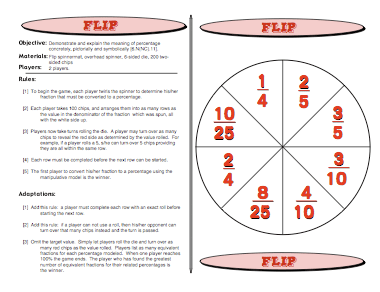(14) Flip

6.RP Understand ratio concepts and use ratio reasoning to solve problems.

Common Core Standards for Mathematical Practice
4. Model with mathematics.

####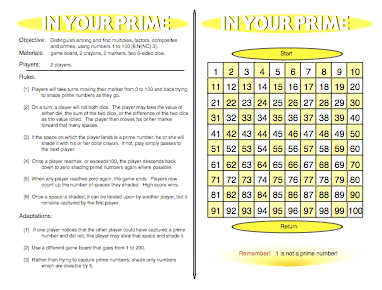(15) In Your Prime

6.NS Compute fluently with multi-digit numbers and find common factors and multiples.

Common Core Standards for Mathematical Practice
5. Use appropriate tools strategically.

####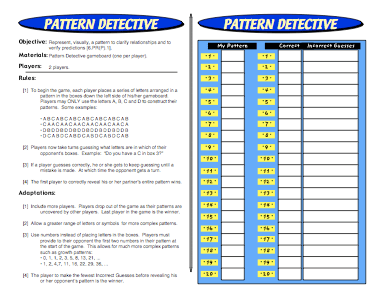(16) Pattern Detective

5.OA Analyze patterns and relationships.

Common Core Standards for Mathematical Practice
7. Look for and make use of structure.

####(17) Not So Common Multiples

6.NS Compute fluently with multi-digit numbers and find common factors and multiples.

####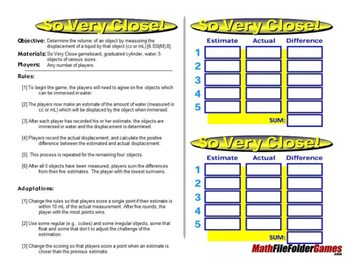(18) So Very Close!

3.MD Solve problems involving measurement and estimation of intervals of time, liquid volumes, and masses of objects.

5. MD Geometric measurement: understand concepts of volume and relate volume to multiplication and to addition.

6. G Solve real-world and mathematical problems involving area, surface area, and volume.

####(19) Tangled Angles

4.G Draw and identify lines and angles, and classify shapes by properties of their lines and angles.

####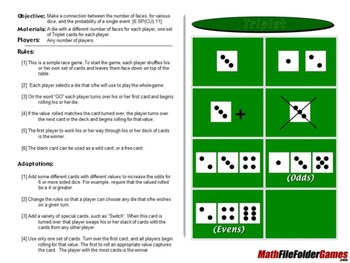(20) Triplet

7.SP Investigate chance processes and develop, use, and evaluate probability models.

Common Core Standards for Mathematical Practice
4. Model with Mathematics

####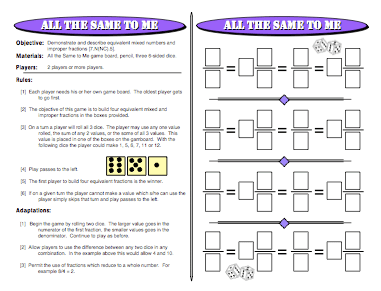(21) All the Same to Me

3.NF Develop understanding of fractions as numbers.

4.NF Extend understanding of fraction equivalence and ordering.

####(22) Above ‘n Below Battleship

5.G Graph points on the coordinate plane to solve real-world and mathematical problems.

8.F Define, evaluate, and compare functions.

####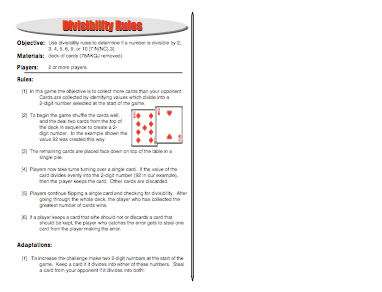(23) Divisibility Rules

4.OA Gain familiarity with factors and multiples.

6.NS Compute fluently with multi-digit numbers and find common factors and multiples.

####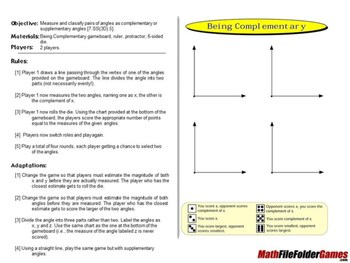(24) Being Complementary

4.G Draw and identify lines and angles, and classify shapes by properties of their lines and angles.

Common Core Mathematical Practices
6. Attend to precision.

####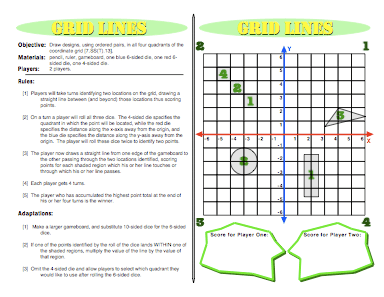(25) Grid Lines

5.G Graph points on the coordinate plane to solve real-world and mathematical problems.

####(26) Percentage Snap

6.RP Understand ratio concepts and use ratio reasoning to solve problems.

####(27) Integer Addition

7.NS Apply and extend previous understandings of operations with fractions to add, subtract, multiply, and divide rational numbers.

Common Core Standards for Mathematical Practice
4. Model with mathematics.

####(28) Power Roller

6.EE.1 Apply and extend previous understandings of arithmetic to algebraic expressions.
1. Write and evaluate numerical expressions involving whole-number exponents.

####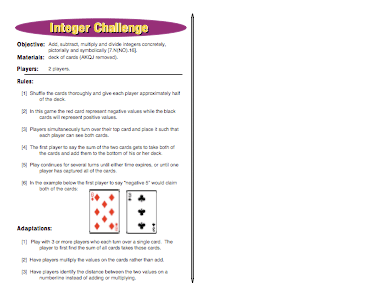(29) Integer Challenge

7.NS Apply and extend previous understandings of operations with fractions to add, subtract, multiply, and divide rational numbers.

Common Core Standards for Mathematical Practice
4. Model with mathematics.

####(30) Switch

6.NS Apply and extend previous understandings of numbers to the system of rational numbers.

7. Understand ordering and absolute value of rational numbers.

####(31) Tangram Chess

8.G Understand congruence and similarity using physical models, transparencies, or geometry software.

####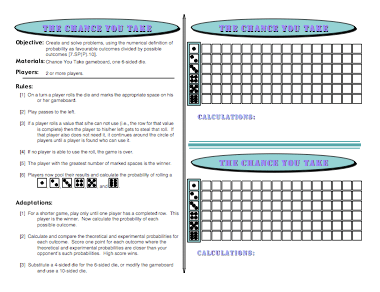(32) The Chance You Take

7.SP Investigate chance processes and develop, use, and evaluate probability models.

Common Core Standards for Mathematical Practice
4. Model with Mathematics

####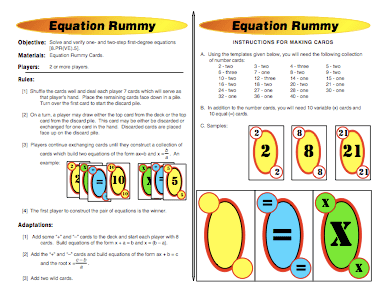(33) Equation Rummy

6.EE Reason about and solve one-variable equations and inequalities.
7. Solve real-world and mathematical problems by writing and solving equations of the form x + p = q and px = q for cases in which p, q and x are all nonnegative rational numbers.

Common Core Standards for Mathematical Practice
4. Model with Mathematics

####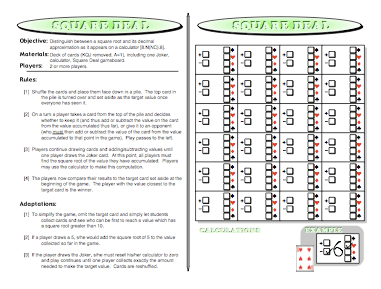(34) Square Deal

8.EE Work with radicals and integer exponents.

####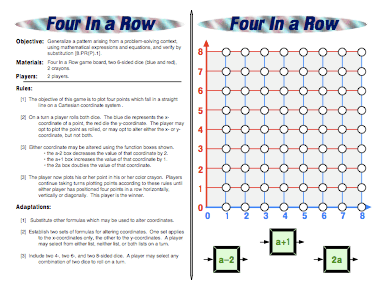(35) Four in a Row

4.OA Generate and analyze patterns.

5.G Graph points on the coordinate plane to solve real-world and mathematical problems.

7.EE Solve real-life and mathematical problems using numerical and algebraic expressions and equations.

####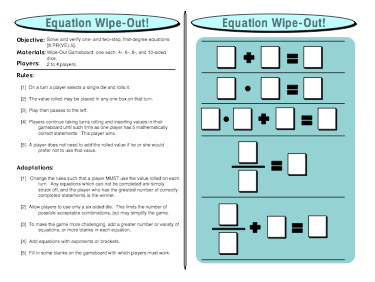(36) Equation Wipe-Out

6.EE Reason about and solve one-variable equations and inequalities.
7. Solve real-world and mathematical problems by writing and solving equations of the form x + p = q and px = q for cases in which p, q and x are all nonnegative rational numbers.

####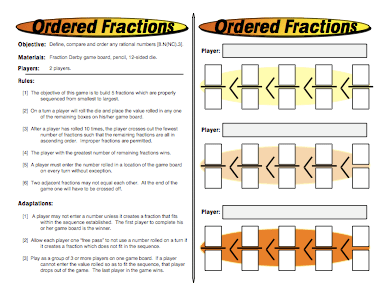(37) Ordered Fractions

3.NF Develop understanding of fractions as numbers.

4.NF Extend understanding of fraction equivalence and ordering.

####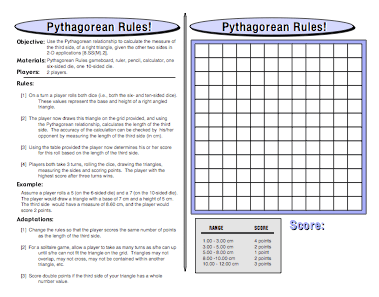(38) Pythagorean Rules

8.G Understand and apply the Pythagorean Theorem.
7. Apply the Pythagorean Theorem to determine unknown side lengths in right triangles in real-world and mathematical problems in two and three dimensions.
8. Apply the Pythagorean Theorem to find the distance between two points in a coordinate system.

Common Core Mathematical Practices
6. Attend to precision.

####(39) Substitution Cross-Off

5.G Graph points on the coordinate plane to solve real-world and mathematical problems.

6.EE Apply and extend previous understandings of arithmetic to algebraic expressions.

Reason about and solve one-variable equations and inequalities.
7. Solve real-world and mathematical problems by writing and solving equations of the form x + p = q and px = q for cases in which p, q and x are all nonnegative rational numbers.

####(40) The Mean Game

6.SP Develop understanding of statistical variability.

####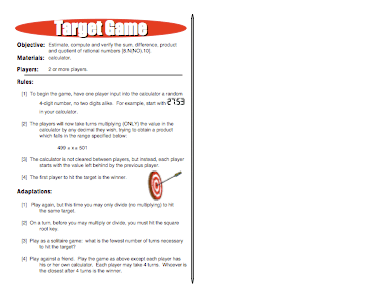(41) Target Game

6.RP Understand ratio concepts and use ratio reasoning to solve problems.

6.NS Apply and extend previous understandings of numbers to the system of rational numbers.

####(42) Unlucky Zeros

7.NS Apply and extend previous understandings of operations with fractions to add, subtract, multiply, and divide rational numbers.

# Get Your Copy of all 42 Middle School Math Games

## A Deeper Look at a Couple of the Math Games

### Entrapment: Transformation Math Games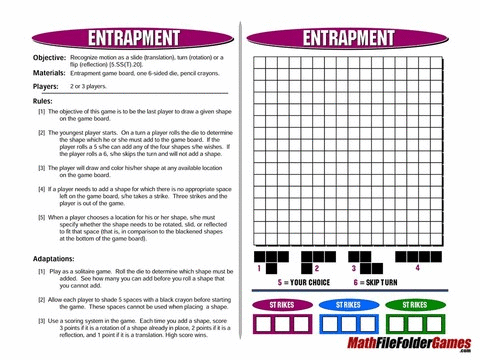Download this Game FREE and File it Away for Later (Click on the PREVIEW Button)!

This visually challenging game helps students understand congruence through translation (slides), rotations (turns), or reflections (flips). At the beginning, when the board is completely open and no squares have been filled in the game doesn’t appear to be too difficult. After all, we’re just filling in patterns of squares, right? Well, as players keep filling in the board, it’s more and more challenging to find ways to slide, turn, and flip the available shapes to fit the remaining blank squares. Some of the most mind-bending are the rotations. They’re not obvious at all and it sometimes takes quite a bit of mental gymnastics to figure out if something really will fit.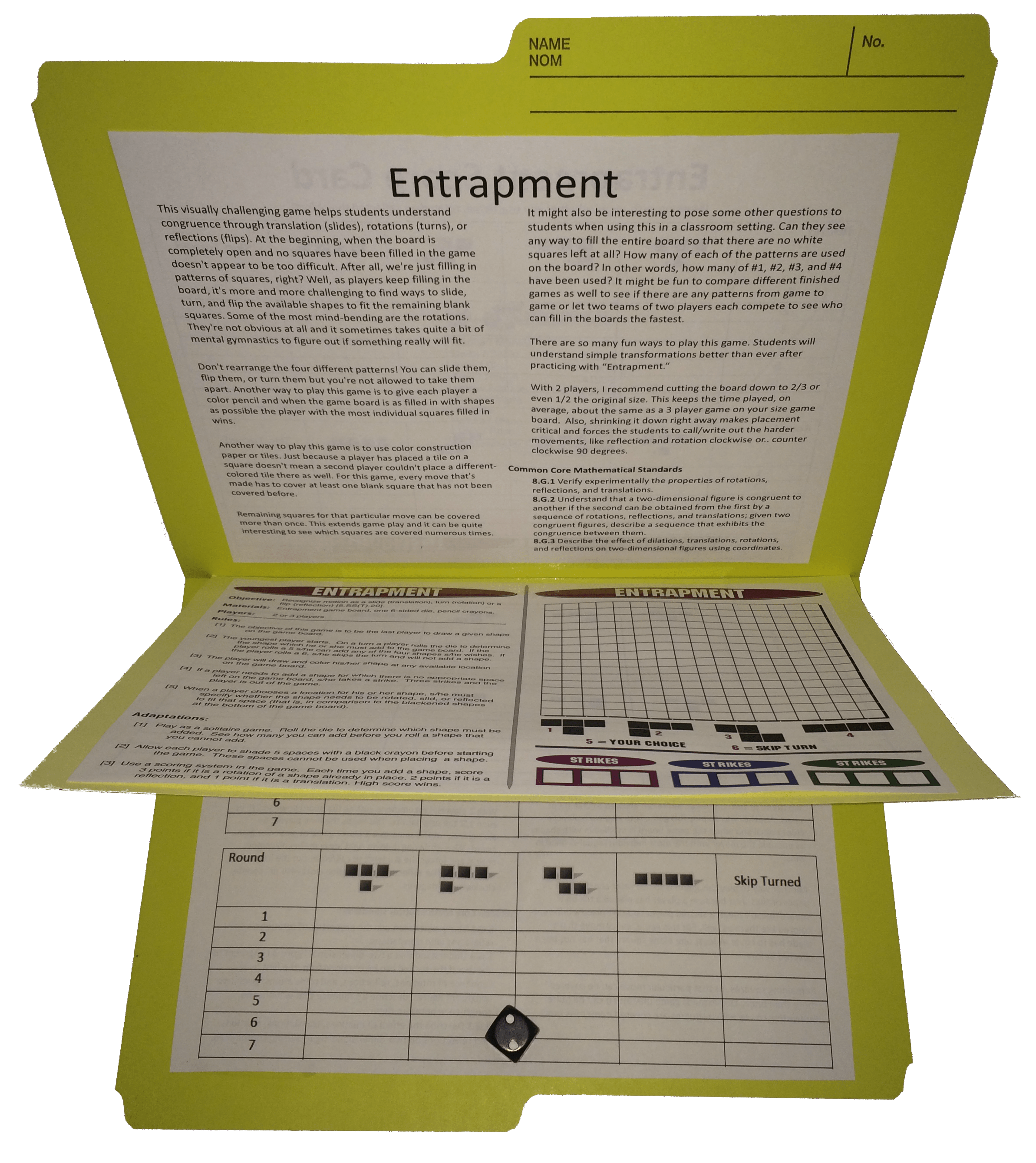Don’t rearrange the four different patterns! You can slide them, flip them, or turn them but you’re not allowed to take them apart. Another way to play this game is to give each player a color pencil and when the game board is as filled in with shapes as possible the player with the most individual squares filled in wins.

Another way to play this game is to use color construction paper or tiles. Just because a player has placed a tile on a square doesn’t mean a second player couldn’t place a different-colored tile there as well. For this game, every move that’s made has to cover at least one blank square that has not been covered before. Remaining squares for that particular move can be covered more than once. This extends game play and it can be quite interesting to see which squares are covered numerous times.

It might also be interesting to pose some other questions to students when using this in a classroom setting. Can they see any way to fill the entire board so that there are no white squares left at all? How many of each of the patterns are used on the board? In other words, how many of #1, #2, #3, and #4 have been used? It might be fun to compare different finished games as well to see if there are any patterns from game to game or let two teams of two players each compete to see who can fill in the boards the fastest.

There are so many fun ways to play this game. Students will understand simple transformations better than ever after practicing with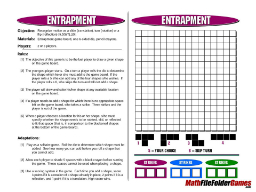#### Common Core Mathematical Standards

8.G.1 Verify experimentally the properties of rotations, reflections, and translations.
8.G.2 Understand that a two-dimensional figure is congruent to another if the second can be obtained from the first by a sequence of rotations, reflections, and translations; given two congruent figures, describe a sequence that exhibits the congruence between them.
8.G.3 Describe the effect of dilations, translations, rotations, and reflections on two-dimensional figures using coordinates.

“I see this as an excellent source for higher-order thinking. I will be using this during an Observation knowing it will be the “hook” my students need to engage in their understanding of new knowledge! Thank you! Great idea!” – CATHERINE P.

“My students had fun playing this game!” – Anette K.

“Easy to use – my kids loved it!” – Ashley Seehusen

“Thanks for making such a fantastic product! It has been very useful in my resource room!” – David H.

“I am looking forward to using this with my students in a few weeks; thank you!” – Jennifer L.

“My students really enjoy this game!” – Denise S.

“Students loved this!” – Sarah H.

“What a fun way to use these skills! Thanks for sharing this freebie – from another Alberta teacher!” – Karen C.

“This game is pretty fabulous!” – Celestine Van Rensselaer

“Your 5 minute filler games are great for end of term maths to do stations with. Kids love them and so do I – Thank-you so much!!” – Anne G.# Get Your Copy of all 42 Middle School Math Games

### Factors: Multiples, Factors, Composites and Primes GameThis fun Factors Game helps kids to more fully understand what prime factorization is all about. By systematically finding the factors of different numbers, kids will see the prime numbers magically appear. This game helps kids connect the meaning of prime numbers, composite numbers, greatest common factor, prime factorization, and least common multiple. There are many different types of strategies you can use to adapt the game. One strategy is to have one student do evens and another do odds.

Numbers like 60 that have a lot of factors will become more practiced in students’ minds after playing this game. Another way to play the game is to use dimes or something that will cover the numbers and then seeing how many dimes end up on a square. In other words, how many times will the number “3” be a factor for the different numbers in this chart. You can also tie the rules of divisibility to this game so that students can think about whether numbers such as 2, 3, or 5 are factors of a specific number.

If you have a lot of different students you can assign them each a number from the game and once they play they can decide whether their assigned number makes them a “prime” or “composite.” Challenge students to see if there are rows and columns they can dismiss as composites by using the divisibility rules.

When using this game in a traditional classroom setting, you can connect the different methods for finding prime factorization, such as factor trees, to the factors on the game board.

In addition to giving students ample time to practice factorization, this game allows them to use problem-solving strategies and patterns to beat their opponents. Students learn about prime factorization and have fun at the same time!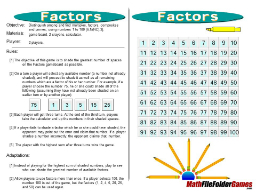The Factors Game Meets Common Core Mathematical Standard
6.NS.4 – Compute fluently with multi-digit numbers and find common factors and multiples.

Common Core Standards for Mathematical Practice
1. Make sense of problems and persevere in solving them.
7. Look for and make use of structure.